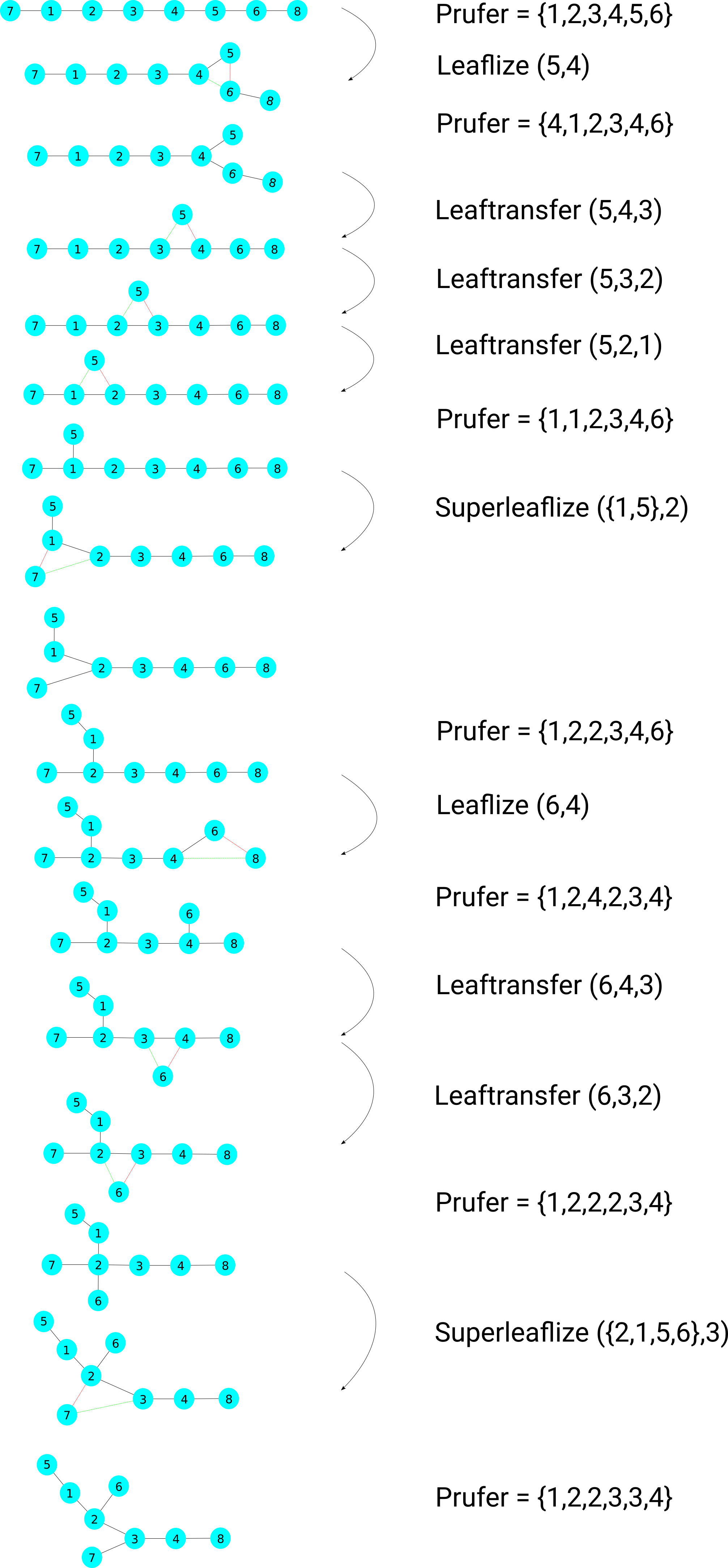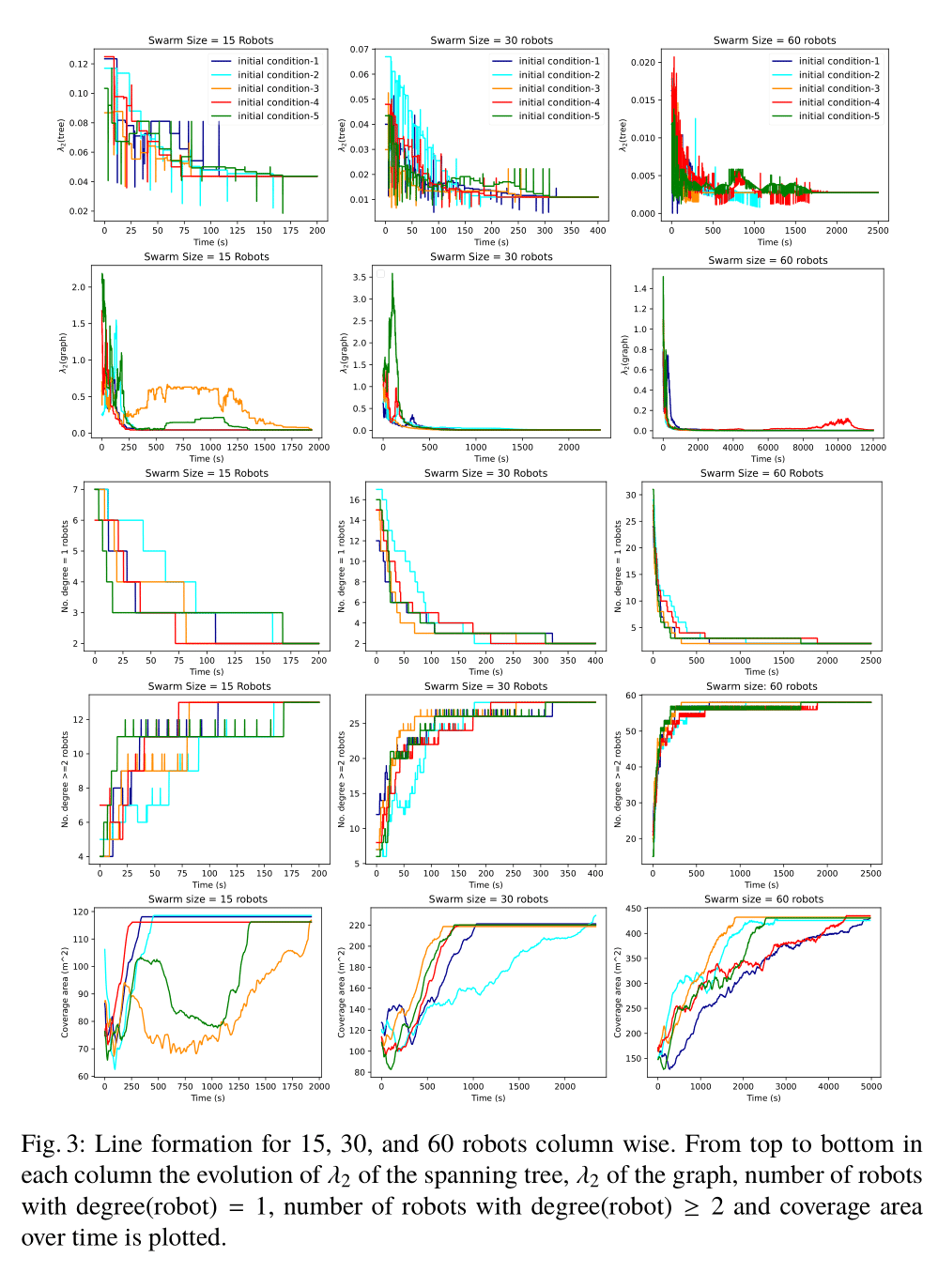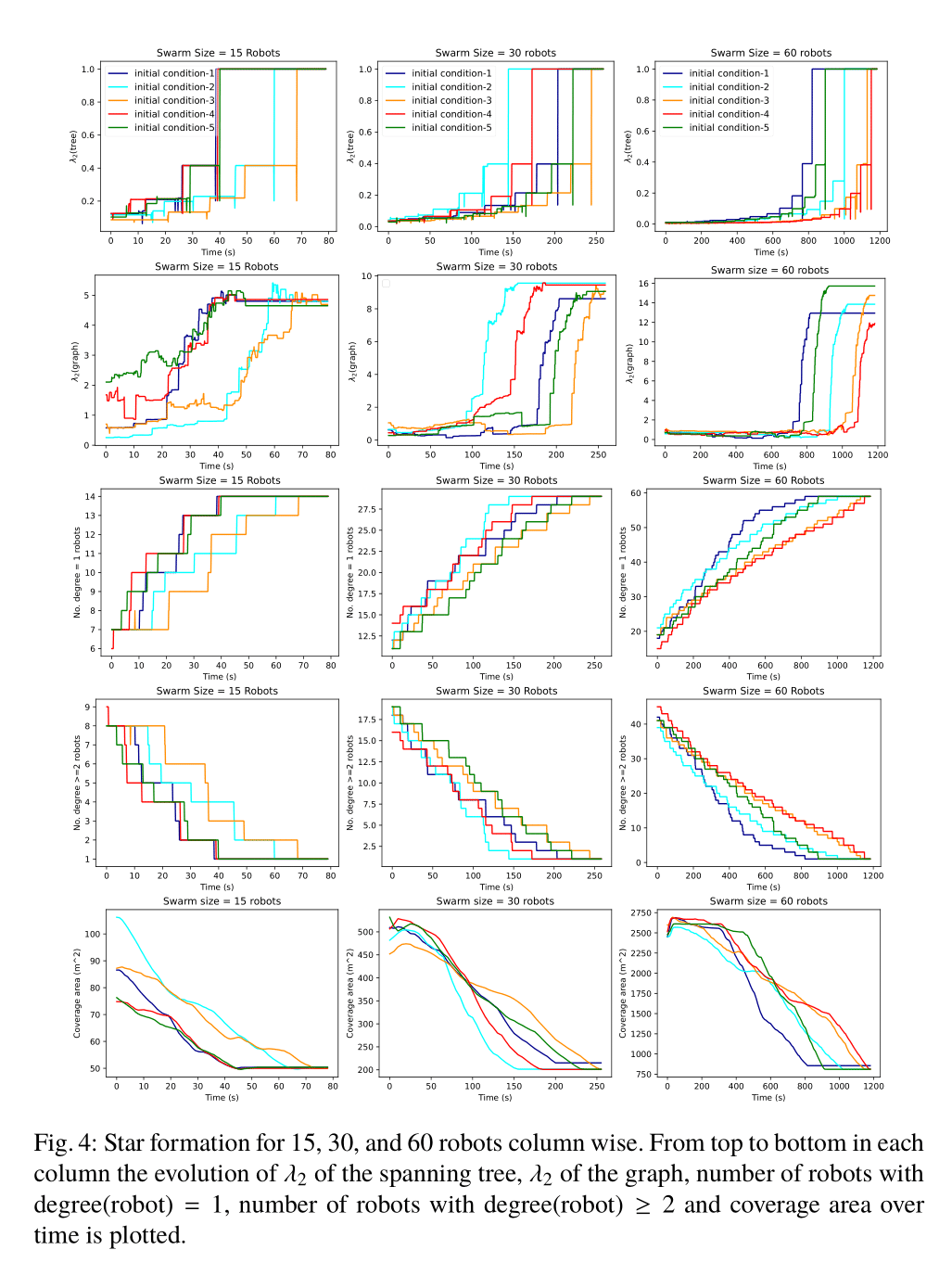# Supplementary Material

We provide extra information for the experiments and results in the paper.

## Decentralised rules for robots swarms to form Line and Star

### 1. Line formation

The line topology is a special topology, where all the robots have either one neighbor (the ends of the line) and all other robots have two neighbors. The rules that the robots have to follow to form a line are:

• Robots with degree(robot)>2 to be called Leaf transferer will do a random leaf transfer or super leaf transfer operation. For this they need at least two of their neighbors to be free (i.e., not involved in any other operation). To avoid getting caught in loops the leaf/super leaf remembers the previous leaf transferer and passes this information to the current leaf transferer. The leaf transferer, therefore, doesn’t transfer the leaf or super leaf to the previous leaf transferer.

• Robots with exactly two neighbors try to straighten the angle between their neighbors by moving towards a direction so as to reduce the obtuse angle.

The parameters used for line formation experiment are Rtransfer = 1m, Rmission = 1.5m, Rrange = 2.5m.

### 2. Star formation

We consider a single root star formation. In star topology, all the robots except the root have one neighbor and the root has N − 1 neighbors. The rules that the robots have to follow to form a line are:

• Each robot connected to the root with more than one neighbor is called a leaflizer, which leaflizes with the root by transferring all its neighbors to the root.
• The root calculates the angles between its leaves and informs them about the direction to make these angles equal.

This table shows the parameters used in the star formation experiments.

 No of robots Rrange(m) Rtransfer(m) RMission 15 2.5 1 1.5 30 5 2 3 60 10 4 6

## Verification of Theorem and Lemma

### An example for conversion from one tree to another tree with Prufer Sequence

This shows an example as to how to use the prufer sequences and the operations to converrt from any initial tree to final tree.### test_theorem.ipynb

In order to verify transformation of trees, we implemented a script in python which verifies the conversion of all possible topologies for a swarm of size 10 to a specific random topology. We also verify that from a random topology it is possible to convert to all other possible topologies.

def sequence_output(input_prufer,final_prufer,plot=False):

A_input,input_leaves = visualizeP2A(input_prufer,plot=plot,title="Input")
A_final,final_leaves = visualizeP2A(final_prufer,plot=plot,title="Output")

i = 0
for i in range(len(input_prufer)):
if i == 0:
if not is_leaf(A_input,final_leaves[i]-1): #if the first leaf of final tree is not a leaf
neighbour = np.nonzero(A_input[final_leaves[i]-1])
#if the first leaf of the final tree is not a leaf in the first leaf leaflize it.
temp_a = leaflize(A_input,final_leaves[i]-1,neighbour)
title = "Leaflize: "+str(final_leaves[i])+" with: "+str(neighbour+1)
_ = visualizeA2P(temp_a,prufer_flag=True,plot=plot,title=title)

if temp_a[final_leaves[i]-1][final_prufer[i]-1] == 0: #if the created leaf is not connected to the final prufer do a leaf transfer.
neighbour = np.nonzero(temp_a[final_leaves[i]-1])
temp_a = leaftransfer(temp_a,neighbour,final_leaves[i]-1,final_prufer[i]-1)
title = "Leaftransfer: leaf: "+str(final_leaves[i])+" from: "+str(neighbour+1)+" to: "+str(final_prufer[i])
_ = visualizeA2P(temp_a,prufer_flag=True,plot=plot,title=title)

elif is_leaf(A_input,final_leaves[i]-1): #if it is a leaf
if A_input[final_leaves[i]-1][final_prufer[i]-1] == 0: #if it is not connected to final prufer do a leaf transfer
neighbour = np.nonzero(A_input[final_leaves[i]-1])
temp_a = leaftransfer(A_input,neighbour,final_leaves[i]-1,final_prufer[i]-1)
title = "Leaftransfer: leaf: "+str(final_leaves[i])+" from: "+str(neighbour+1)+" to: "+str(final_prufer[i])
_ = visualizeA2P(temp_a,prufer_flag=True,plot=plot,title=title)

else: # good to proceed
temp_a = np.copy(A_input)

elif i>0:
if is_leaf(temp_a,final_leaves[i]-1):
if temp_a[final_leaves[i]-1][final_prufer[i]-1] == 0: #if the leaf is not connected to the final prufer do a leaf transfer.
neighbour = np.nonzero(temp_a[final_leaves[i]-1])
temp_a = leaftransfer(temp_a,neighbour,final_leaves[i]-1,final_prufer[i]-1)
title = "Leaftransfer: leaf: "+str(final_leaves[i])+" from: "+str(neighbour+1)+" to: "+str(final_prufer[i])
_ = visualizeA2P(temp_a,prufer_flag=True,plot=plot,title=title)

elif not is_leaf(temp_a,final_leaves[i]-1):
all_neighbours = np.nonzero(temp_a[final_leaves[i]-1])
neighbours = np.setdiff1d(all_neighbours,np.subtract(final_leaves[0:i],np.ones(len(final_leaves[0:i]),dtype=int)))
branches_to_keep = np.intersect1d(all_neighbours,np.subtract(final_leaves[0:i],np.ones(len(final_leaves[0:i]),dtype=int)))
if len(neighbours) == 1: # the subtree is a leaf
if temp_a[final_leaves[i]-1][final_prufer[i]-1] == 0: #if the created leaf is not connected to the final prufer do a leaf transfer.
neighbour = neighbours
temp_a = leaftransfer(temp_a,neighbour,final_leaves[i]-1,final_prufer[i]-1)
title = "Leaftransfer: leaf: "+str(final_leaves[i])+" from: "+str(neighbour+1)+" to: "+str(final_prufer[i])
_ = visualizeA2P(temp_a,prufer_flag=True,plot=plot,title=title)

elif len(neighbours) > 1: #leaflize the superleaf
temp_a = leaflize(temp_a,final_leaves[i]-1,neighbours,branches_to_keep)
title = "Leaflize: "+str(final_leaves[i])+" with: "+str(neighbours+1)
_ = visualizeA2P(temp_a,prufer_flag=True,plot=plot,title=title)

if temp_a[final_leaves[i]-1][final_prufer[i]-1] == 0: #if the created leaf is not connected to the final prufer do a leaf transfer.
neighbour = neighbours
temp_a = leaftransfer(temp_a,neighbour,final_leaves[i]-1,final_prufer[i]-1)
title = "Leaftransfer: leaf: "+str(final_leaves[i])+" from: "+str(neighbour+1)+" to: "+str(final_prufer[i])
_ = visualizeA2P(temp_a,prufer_flag=True,plot=plot,title=title)

if i == len(input_prufer)-1:
P = visualizeA2P(temp_a,prufer_flag=True,plot=False)
if (P == final_prufer):
print("input_prufer: " + str(input_prufer) + " final_prufer: "+ str(final_prufer)+" success: "+"True")
return True
else:
print("input_prufer: " + str(input_prufer) + " final_prufer: "+ str(final_prufer)+" success: "+"False")
return False


### test_theorem_line_star.ipynb

We also have verified this algorithm in a python script where starting from any random topology we were able to form a star/line for a swarm of size 10 for all the possible 108 combinations.

def line(input_prufer,plot=False):

temp_a,_ = visualizeP2A(input_prufer,plot=plot,title="Input")
num_neighbours = np.matmul(temp_a,np.ones((temp_a.shape,1))).reshape(-1)
prev_leaf_transfer = np.ones(temp_a.shape)*-1

while np.count_nonzero(num_neighbours > 2)>0:
leaf_transferers = np.argwhere(num_neighbours>=3).reshape(-1)
np.random.shuffle(leaf_transferers)

for leaf_transferer in leaf_transferers:
neighbours = np.argwhere(temp_a[leaf_transferer]==1).reshape(-1)
np.random.shuffle(neighbours)
leaf = neighbours
to_exclude = np.array([leaf,prev_leaf_transfer[leaf]])
neighbours_to_transfer = np.setdiff1d(neighbours,to_exclude)
np.random.shuffle(neighbours_to_transfer)
neighbour_to_transfer = neighbours_to_transfer
prev_leaf_transfer[leaf] = leaf_transferer
temp_a = leaftransfer(temp_a,leaf_transferer,leaf,neighbour_to_transfer)
_ = visualizeA2P(temp_a,prufer_flag=True,plot=plot,title=title)

num_neighbours = np.matmul(temp_a,np.ones((temp_a.shape,1))).reshape(-1)

return np.matmul(temp_a,np.ones((temp_a.shape,1))).reshape(-1)


def star(root,input_prufer,plot=False):
root = root
input_prufer = input_prufer
n = len(input_prufer) + 2
temp_a,_ = visualizeP2A(input_prufer,plot=plot,title="Input")
neighbours_root = np.argwhere(temp_a[root]==1).reshape(-1)

while len(neighbours_root) < n - 1:
for neighbour in neighbours_root:
if not is_leaf(temp_a,neighbour):
title = "leaflize: " + str(neighbour+1) + " with " + str(root+1)
temp_a = leaflize(temp_a,neighbour,root)
visualizeA2P(temp_a,prufer_flag=True,plot=plot,title=title)
neighbours_root = np.argwhere(temp_a[root]==1).reshape(-1)

return np.matmul(temp_a,np.ones((temp_a.shape,1))).reshape(-1)


## Supplementary plots for the experimentsFigures 3 and 4 depict λ2, coverage area, and progress of operations. We plot the time evolution of λ2 of the graph and the maintained spanning tree, as a connectivity index and a parameter specifying consensus rate. The evolution of the number of nodes with degree(robot) = 1 (i.e., having only one neighbor) and degree(robot) = 2 is sketched for the line formation and the evolution of the number of nodes with degree(robot) = 1 and nodes with degree(robot) ≥ 2 for the star formation, which is a progress index in each case. The λ2 of the manipulated spanning tree and the whole graph examine the connectivity awareness of our method which has to stay greater than zero over the experiment. In the specific case of line, λ2 of the tree reduces with time and reaches a constant value when the line has been straightened out. Also, λ2 of the graph will approach the same value, if Rmission is close to Rrange, which is the minimum for a given connected graph of N nodes. However, In the case of the star topology, λ2 of the tree increases to a constant value of one at the end of the experiment no matter the number of nodes in the system, and the λ2 of the graph increases. If Rmission < Rrange/2 it would have approached an all to all graph which has the maximum λ2 for a given connected graph of N nodes. The coverage area has been shown to decrease for the star and to increase for the line case which is showing the trade-off between λ2 and the coverage area and that is why topology manipulation is needed to provide flexibility. Furthermore, for the star formation, we have plotted the number of nodes with degree(robot) = 1 and the number of nodes with degree(robot) ≥ 2. This is to show that the manipulation operations are changing the topology closer to the star topology with time. The number of nodes with degree(robot) = 1 for the star case increases to N − 1 and there is exactly one node that has N − 1 neighbors, which is the root. The plots show the evolution of these metrics which is increasing for the number of nodes with degree(robot) = 1 and decreasing for the number of nodes with degree(robot) ≥ 2. For line formation, we have shown a similar metric that reduces to 2 for the number of nodes degree(robot) = 1 and increases to N − 2 for the number of nodes degree(robot) = 2 which is the definition of a line topology.

## Setting up the simlations for Line and Star in Argos3 and Buzz.

The code for the experiments can be found here. ARGoS3 Simulator ARGoS3 simulator can also be installed from binaries please refer to the official website for more information: https://www.argos-sim.info/ The instructions below are for installing ARGoS3 from its source. Official code repository: https://github.com/ilpincy/argos3 Dependencies for ARGoS3 can be installed using the following command:

sudo apt-get install cmake libfreeimage-dev libfreeimageplus-dev \
qt5-default freeglut3-dev libxi-dev libxmu-dev liblua5.3-dev \
lua5.3 doxygen graphviz graphviz-dev asciidoc

Installations for Argos3

$git clone https://github.com/ilpincy/argos3.git argos3$ cd argos3
$mkdir build_simulator$ cd build_simulator
$cmake ../src$ make
$sudo make install Installations for Buzz $ cd Buzz
$mkdir build$ cd build
$cmake ../src$ sudo make install
$sudo ldconfig Installations for Khepera V $ git clone https://github.com/ilpincyargos3-kheperaiv.git
$mkdir build_sim$ cd build_sim
$cmake -DCMAKE_BUILD_TYPE=Release ../src$ make
$sudo make install Building the loop function for ARGoS: $ cd files/loop_fun_src/
$mkdir build$ cd build/
$cmake ..$ make

Building the buzz script.

$cd buzz_scripts$ bzzc leaf_transfer2.bzz/leaflize_15.bzz/leaflize_30.bzz/leaflize_60.bzz
# for line/star_15/star_30/star_60
To run the Argos3 file.
argos3 -c files/star.argos # for 15 robots
argos3 -c files/line.argos # for 15 robots